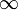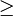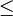12-240/Classnotes for Thursday October 11

In this lecture, the professor concentrate on corollaries of basic and dimension.

Annoucements

TA Office Hours (Still pending!) @ 215 Huron St., 10th floor.

Peter - 11am - 1pm

Brandon 1pm - 3pm

Topic: Replacement Theorem

corollaries

1/ If V has a finite basic β1, then any other basic β2 of V is also finite and |β1|=|β2|

2/ "dim V" makes sense

dim V = |β| if V has a finite basic β

Otherwise, dim V =$\infty \!\,$

ex: dim P(F)=$\infty \!\,$

3/ Assume dim V = n <$\infty \!\,$ then,

a) If G generate V then |G|$\ge \!\,$ n & some set of G is a basic of V. ( If |G|= n, itself is a basic)

b) If L is linearly independent then |L|$\le \!\,$ n, if |L|=n then L is a basic, if |L|< n then L can be extended to become a basic.

Proofs

1) β2 generate and β1 is linearly independent

From replacement theorem

|β2|$\ge\!\,$ |β1| , ( role reversal), |β1|$\ge\!\,$ |β2|

Then |β2|= |β1|

3) a) (|G|$\ge\!\,$ n)

by dim V = n, exist basic β of V with n elements, Take L = β in the replacement lemma, |G| = n1

|L|$\le\!\,$ n1= |G|

Hence n$\le\!\,$ |G|